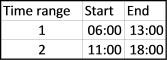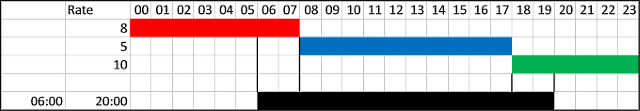Author: Oscar Cronquist Article last updated on March 26, 2018

I found an old post that I think is interesting to write about today. Think of two overlapping ranges, it may be dates, times or whatever, the formula demonstrated here works with everything.

This picture shows two time ranges, 06:00-13:00 (yellow) and 11:00-18:00 (green).It is obvious that there are two overlapping hours but how do we calculate it in excel?

The MEDIAN function comes to rescue, but first let me explain the function. It returns a value that separates the higher half of a data set from the lower half. Example, MEDIAN(1,2,3) returns 2. 1 is the lower half and 3 is the higher half.

MEDIAN(1,2,3,4,5,6) returns 3.5 because there are two values (3, 4) separating the higher half (5,6) from the lower half (1,2). The average of these two values is 3.5.

### Calculate overlapping hours

We have 4 times here to remember, the start and end of time range 1 and 2.Let see what happens if we use the MEDIAN function with the start and end value of time range 1 and only the start value of time range 2.

MEDIAN("06:00", "13:00", "11:00") returns 11:00.

And then the end of time range 2.

MEDIAN("06:00", "13:00", "18:00") returns 13:00

13:00 - 11:00 is 02:00. Two hours are overlapping.

The formula becomes

MEDIAN(Start1,End1,End2) - MEDIAN(Start1,End1,Start2)

and returns 02:00.

### Lets do something more complicated

Between 00:00 and 08:00 the rate is 8, 08:00-18:00 the rate is 5 and 18:00 to 24:00 the rate is 10.How do we calculate total cost if the time range is 06:00-20:00?

Count overlapping hours for the first range 00:00-08:00 and multiply with rate 8.

=HOUR(MEDIAN("06:00", "20:00", "08:00") - MEDIAN("06:00","20:00", "00:00"))*8

returns 16.

Count overlapping hours for the second range 08:00-18:00 and multiply with rate 5.

=HOUR(MEDIAN("06:00", "20:00", "18:00") - MEDIAN("06:00","20:00", "08:00"))*5

returns 50

Count overlapping hours for the second range 18:00-24:00 and multiply with rate 10.

=HOUR(MEDIAN("06:00", "20:00", "24:00") - MEDIAN("06:00","20:00", "18:00"))*10

returns 20Combining all formulas gives

=HOUR(MEDIAN("06:00", "20:00", "08:00") - MEDIAN("06:00","20:00", "00:00"))*8+HOUR(MEDIAN("06:00", "20:00", "18:00") - MEDIAN("06:00","20:00", "08:00"))*5+HOUR(MEDIAN("06:00", "20:00", "24:00") - MEDIAN("06:00","20:00", "18:00"))*10

returns 86.